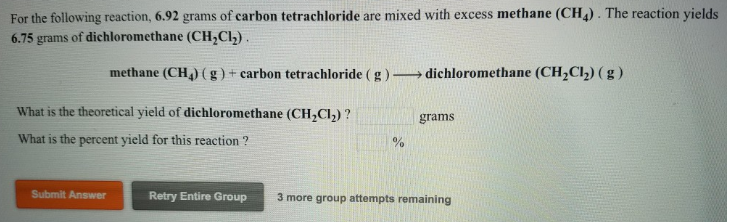# Problem: For the following reaction, 6.92 grams of carbon tetrachloride are mixed with excess methane (CH4). The reaction yields 6.75 grams of dichloromethane (CH2Cl2) methane (CH4) (g) + carbon tetrachloride (g) → dichloromethane (CH2Cl2) (g) What is the theoretical yield of dichloromethane (CH2CI2)? What is the percent yield for this reaction ?

###### FREE Expert Solution
95% (91 ratings)###### Problem Details

For the following reaction, 6.92 grams of carbon tetrachloride are mixed with excess methane (CH4). The reaction yields 6.75 grams of dichloromethane (CH2Cl2

methane (CH4) (g) + carbon tetrachloride (g) → dichloromethane (CH2Cl2) (g)

What is the theoretical yield of dichloromethane (CH2CI2)?

What is the percent yield for this reaction ?